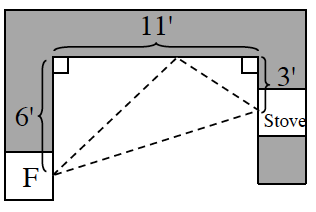Home > CCG > Chapter 7 > Lesson 7.2.6 > Problem7-114

7-114.

After finding out that her kitchen does not conform to industry standards, Lashayia is back to the drawing board (see problem 7‑84). Where can she locate her sink along her top counter so that its distance from the stove and refrigerator is as small as possible? And will this location keep her perimeter below $26$ feet? Show all work. Homework Help ✎This problem is similar to the Interior Design problem from Lesson 7.1.3. How did you find the solution for that problem?

For the shortest possible distance, the two right triangles should be similar.

Set up equivalent ratios of corresponding sides: Remember the side that is length $11$, can be split into two lengths $x$ and $11−x$.

$\frac{6}{x}= \frac{3}{11-x}$

Solve for $x$.